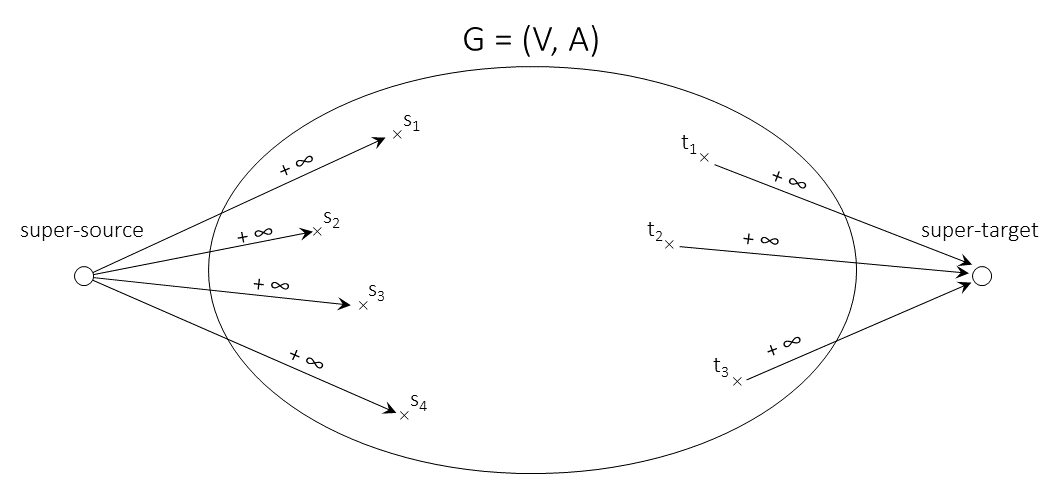# Max-Flow Problems

## Standard version

Input:

1. A directed graph $G=(V,A)$.
2. A source node $s\in V$ and a target (a.k.a. sink) node $t\in V$.
3. A nonnegative upper bound (a.k.a. capacity) $u(a)$ for each arc $a\in A$.

Output: A feasible $(s,t)$-flow that has maximum flow value among all feasible $(s,t)$-flows.

## Generalizations

1. For each arc $a\in A$, there is a lower bound $\ell(a)$, and $f(a)\geq\ell(a)$ is additionally required. The lower bounds need not be nonnegative, so the flow values need not be nonnegative, either. This version is often called maximum flow with edge demands. It may be reduced to solving two instances of the standard version as follows:
1. First, we construct a new graph $G'=(V',A')$ as follows: We add a super-source $s'$ and a super-target $t'$ to $V$. Next, for every node $v\in V$, we add an arc $(s',v)$ and an arc $(v,t')$ to $A$. Finally we add an arc $(t,s)$ to $A$, if not in $A$ yet.
2. For all arcs $a\in A$, we set $\ell'(a):=0$. For each node $v\in V$, we set
$u'(s',v):=\max\{0,\sum_{(w,v)\in A}\ell(w,v)\}$ and $u'(v,t'):=\max\{0,\sum_{(v,w)\in A} \ell(v,w)\}$.
For all arcs $a\in A$, we set $u'(a):=u(a)-\ell(a)$. Finally, we set $u'(t,s):=+\infty$.
3. If all additional arcs $(s',\cdot)$ and $(\cdot,t')$ are saturated by some feasible (and obviously maximum) $(s',t')$-flow $f$, the values $f(a)+\ell(a)$ on all original arcs of $G$ obviously form a feasible $(s,t)$-flow in $G$ with respect to $\ell$ and $u$. On the other hand, if there is a feasible $(s,t)$-flow $f$ in $G$ with respect to $\ell$ and $u$, the values $f(a)-\ell(a)$ on all original arcs in $G$ along with saturating flow values on all additional arcs form a feasible (and obviously maximum) $(s',t')$-flow.
4. Therefore, we may safely terminate the whole procedure if the additional arcs are not saturated by a maximum $(s',t')$-flow. Otherwise, we may construct an instance $(G'=(V,A'),s,t,u')$ of the standard version as follows: for each original arc $a=(v,w)\in A$ insert an opposite arc $a'=(w,v)$ and set $u'(a):=u(a)-f(a)\geq 0$ and $u'(a'):=f(a)-\ell(a)\geq 0$ (cf. residual network).Max-Flow Problem with several sources and targets
2. More than one source and more than one target can be reduced to the standard version by adding a super-source node $s$, a super-target node $t$, an arc $(s,v)$ with "infinite" capacity for each source $v$, and an arc $(v,t)$ with "infinite" capacity for each target $v$ (for example, the sum of the upper bounds of all arcs is sufficiently large to serve as "infinity").
3. Usually, the term generalized flow is reserved for the specific generalization in which for each node $v\in V\setminus\{s,t\}$, the ratio of the total sum of all incoming flow and the total sum of all outgoing flow is given (in the standard version, this ratio is 1 due to the flow conservation condition).
4. The max-flow problem asks for an optimal steady-state flow. However, in many applications, a certain amount of flow is to be sent as soon as possible from the source to the target. It is easy to see that, if the amount of flow is sufficiently large, then an optimal solution is constant most of the time, and the maximum steady-state flow is this constant flow.